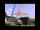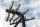Triangle

Calculate the sides of the triangle if its area S = 630 and the second cathethus is shorter by 17.

Result

a =  45
b =  28
c =  53

Solution:Try calculation via our triangle calculator.

Leave us a comment of this math problem and its solution (i.e. if it is still somewhat unclear...):Be the first to comment!To solve this verbal math problem are needed these knowledge from mathematics:

Looking for help with calculating roots of a quadratic equation? Do you have a linear equation or system of equations and looking for its solution? Or do you have quadratic equation? Pythagorean theorem is the base for the right triangle calculator.

Next similar math problems:

1. Rectangular trapezoidCalculate the content of a rectangular trapezoid with a right angle at the point A and if |AC| = 4 cm, |BC| = 3 cm and the diagonal AC is perpendicular to the side BC.
2. CathetiThe hypotenuse of a right triangle is 41 and the sum of legs is 49. Calculate the length of its legs.
3. A mastA mast 32 meters high was broken by the wind so that its top touches the ground 16 meters from the pole. The still standing part of the mast, the broken part and the ground form a rectangular triangle. At what height was the mast broken?
4. Proof PTCan you easy prove Pythagoras theorem using Euclidean theorems? If so, do it.
5. ColumnPerpendicular pole high 8 m tall broke and its toe fell 2.7 m from the bottom of the pole. At what height above the ground pole broke?
6. Truncated cone 5The height of a cone 7 cm and the length of side is 10 cm and the lower radius is 3cm. What could the possible answer for the upper radius of truncated cone?
7. 4s pyramidRegular tetrahedral pyramid has a base edge a=17 and collaterally edge length b=32. What is its height?
8. DistanceWha is the distance between the origin and the point (18; 22)?
9. RTriangle 17The hypotenuse of a right triangle is 17 cm. If you decrease both two legs by 3 cm you will reduce the hypotenuse by 4 cm. Determine the length of this legs.
10. RootsDetermine the quadratic equation absolute coefficient q, that the equation has a real double root and the root x calculate: ?
11. EquationEquation ? has one root x1 = 8. Determine the coefficient b and the second root x2.
12. Solve 3Solve quadratic equation: (6n+1) (4n-1) = 3n2
13. DiscriminantDetermine the discriminant of the equation: ?Find the roots of the quadratic equation: 3x2-4x + (-4) = 0.For what x expression ? equals zero?The fifth of the number is by 24 less than that number. What is the number?We want to prove the sentence: If the natural number n is divisible by six, then n is divisible by three. From what assumption we started?